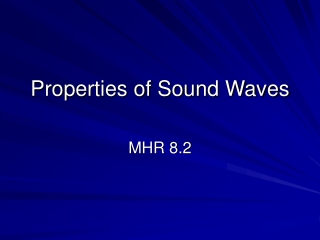Download PresentationProperties of Sound Waves

# Properties of Sound Waves

Télécharger la présentation## Properties of Sound Waves

- - - - - - - - - - - - - - - - - - - - - - - - - - - E N D - - - - - - - - - - - - - - - - - - - - - - - - - - -
##### Presentation Transcript

1. Properties of Sound Waves MHR 8.2

2. The Speed of Sound • The speed of sound through air depends on the density of air and the temperature • v= 331 +0.59 Tair • Where v= speed of sound in air measured in m/s • The constant 0.59 accounts for the density of air • Tair is the temperature in 0C • Please note, the units work out to m/s – read MHR pg. 378

3. Problem #1: the speed of sound • Today, the temperature outside is 4 0C. What is the speed of sound in air? • Answer: • v= 333.36 m/s

4. Problem #2: Find Temperature • If the speed of sound is measured to be 318 m/s, what is the air temperature? • Answer: • -22.1 0C

5. Problem #3: Echo • Going back to question #1, if you were hiking in a canyon and shouted, you may hear an echo. • Lets assume you hear the echo 2.8s later. • How far would the canyon wall be? • Answer: • Δ d =466.7 m

6. Mach Number • Mach number: the ratio of the airspeed of an object to the local speed of sound • M = air speed of object/ local speed of sound

7. Problem #4: Mach number • An aircraft is flying 905 km/h in air at a temperature of – 50.0 0C. Calculate the Mach number. • M = 0.835 • So what does 0.835 mean???

8. Mach Number: some facts • As an air craft approaches the speed of sound, it catches up to the sound waves. • These waves build up to form a shock wave- also known as the sound barrier at Mach one • The shock wave can cause severe damage to the plane and pilot • Chuck Yeager was the first pilot to break the sound barrier in 1947

9. Image of plane breaking sound barrier

10. Your Turn • MHR pg. 382 # 1-6 • MHR pg. 422 # 1,2